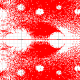One of the most confounding and fundamental open problems in finite geometry is the question of classifying the hyperovals of the Desarguesian projective planes. Beyond this problem, there are many other related interesting avenues of research: what about hyperovals of non-Desarguesian planes, maximal arcs or linear codes with minimum distance 3?

An arc of a projective plane is a set of points with no three lying on a common line. For$q$ odd, the largest an arc can be in the Desarguesian projective plane$PG(2,q)$ is$q+1$, an oval, and it is exhibited by a conic: for example, the solutions to the quadratic equation$XY=Z^2$ (where our points are represented in homoegenous coordinates$(X,Y,Z)$). To see that there can be no larger arc, choose a point$P$ not in the arc; if there were exactly$q+2$ points in the arc, then there would be exactly$(q+2)/2$ lines on$P$ meeting the arc, and this number is not an integer for$q$ odd. A bigger arc would have a sub-arc of size$q+2$, so this argument rules out all larger sizes. For$q$ even, we can have$q+2$ points in an arc, a so-called hyperoval. But then, a similar counting argument shows that there is no larger arc. Segre showed that an oval of$PG(2,q)$ for$q$ odd, must be projectively equivalent to a conic. So the ovals here are completely classified. Does a similar result hold for hyperovals when$q$ is even?

For$q$ even, the tangents of a conic meet in a unique point, the so-called nucleus of the conic. This extra point then completes the conic to a hyperoval. But there’s more!

Bill Cherowitzo’s hyperovals page gives a run-down of the state of the art, what is known about the various constructions of hyperovals (due to Cherowitzo, Lunelli, Sce, Segre, Glynn, O’Keefe, Payne, Penttila). The wikipedia page on ovals is also quite good. By the transitivity on frames of the collineation group of the plane, we can assume that the points$(1,0,0)$,$(0,1,0)$,$(0,0,1)$ and$(1,1,1)$ are contained in any hyperoval. This then implies that there is a function$f$ on$GF(q)$ such that the hyperoval can be represented as$\{(t,f(t),1):t\in GF(q)\}\cup \{(0,1,0,),(1,0,0)\}$. Moreover,$f$ is a permutation and can be written as a polynomial of degree$q-2$. There are some other conditions which$f$ must satisfy, making it a so-called o-polynomial. So we can study o-polynomials to construct and classify hyperovals. For example, Segre showed that for$q=2^h$, with$h$ odd, the polynomial$t^6$ is an o-polynomial, and it gives us a hyperoval which does not come from completing a conic.

The hyperovals of$PG(2,q)$ for$q\le 8$ are known to all arise from a conic (i.e., a hyperconic). For$q=16$ we get a different hyperoval, the Lunelli-Sce example, and according to Marshall Hall Jnr (1975), this together with the hyperconic are the only ones up to equivalence. For$q=32$ and$q=64$ we begin to see the number of inequivalent examples expand, but not by much. For$q=32$, we have a complete classification due to Tim Penttila and Gordon Royle (1994). For$q=64$, we know all of the hyperovals which have nontrivial symmetry (also due to Penttila and Royle, 1995).

For a while now, since Cherowitzo, O’Keefe and Penttila in 2003, we have not seen a new construction of a family of hyperovals in Desarguesian planes. Have we found them all? It is perhaps still too premature to think that we know all of the examples, yet the search for new examples is very difficult in planes of order$128$ or more. I hope to see this field of research come alive again with some beautiful new constructions. Some of the many consequences would be new generalised quadrangles, new partial geometries and new strongly regular graphs.

(Thanks to Tim Penttila for spotting mistakes of mine in a previous draft of this post!)

## 2 thoughts on “Open problems in finite geometry: a classification of hyperovals”

1.Gordon Royle says:
1.Tim Penttila says: